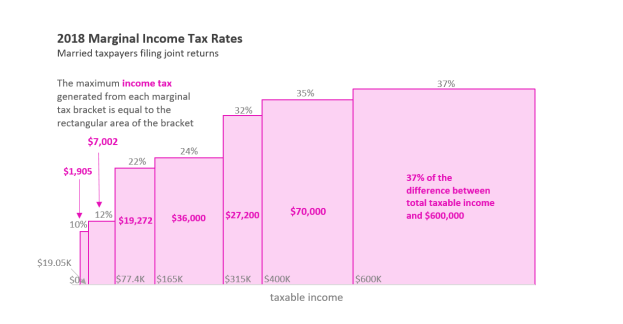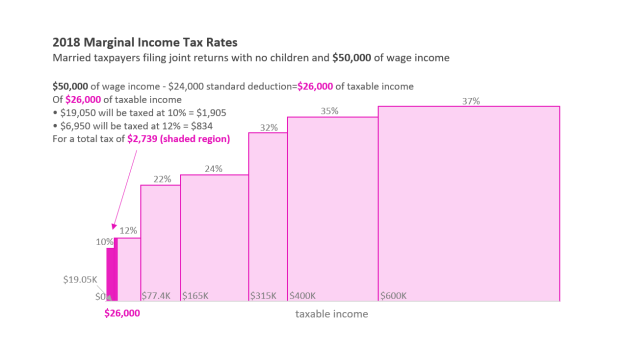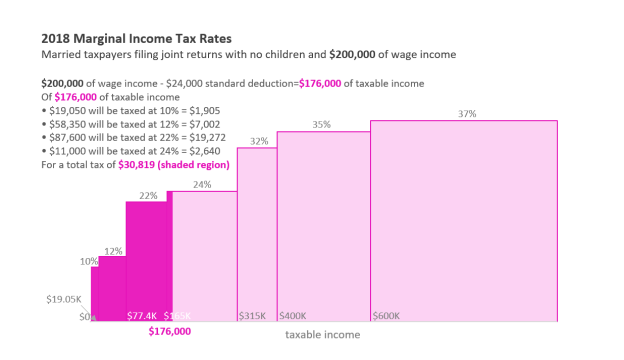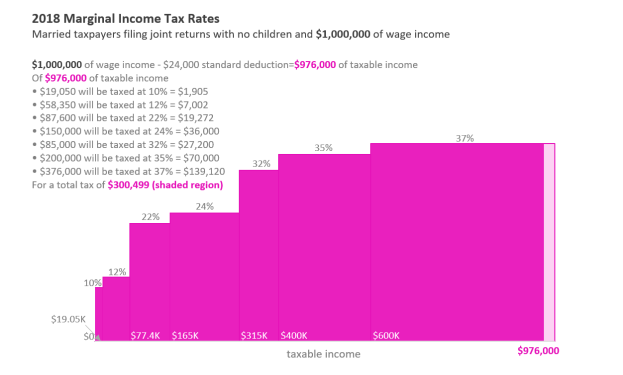# The Federal Income Tax: How Do Marginal Income Tax Rates Work?

## Calculating Income Tax Liability

For many taxpayers, calculating their federal income tax liability can be broken down into three main steps.

• 1. Taxpayers calculate the amount of their income subject to taxation (i.e., their taxable income).
• 2. Taxpayers apply marginal income tax rates to their taxable income to determine their "pre-tax credit" income tax liability.
• 3. Taxpayers subtract any tax credits from their pre-tax credit income tax liability to determine their final income tax liability.

Some taxpayers with more complex tax situations, including those who are subject to the alternative minimum tax (AMT) and those who have income from capital gains and dividends, will have to perform additional steps to calculate their income tax liability. (Those cases are not discussed in this Insight.)

For a visualization of this calculation, see CRS Infographic IG10011, The U.S. Individual Income Tax System, 2018, by Molly F. Sherlock.

## Applying Marginal Income Tax Rates

This Insight focuses on the second step in this process outlined above—applying marginal tax rates to taxable income. For the purposes of this Insight, it will be assumed that the taxpayer has a relatively simple tax situation: they are a married couple with only wage income that claims only one deduction—the standard deduction—and claims no tax credits when calculating their income tax liability. In effect, this couple will need to do only steps one and two outlined above to calculate their income tax liability. For 2018, the standard deduction for a married couple is \$24,000.

(In reality, the calculation of taxable income for a taxpayer may not be so simple and will depend on a variety of factors, including the taxpayer's sources of income, family structure, and eligibility for a variety of deductions—i.e., subtractions from their income.)

Once a taxpayer has calculated their taxable income, they then apply the marginal tax rates to their taxable income. There are currently seven marginal tax rates, and the income ranges over which they apply ("tax brackets") differ based on the taxpayer's filing status; see Table 1 below. The federal income tax is considered a progressive tax by economists because as taxable income increases, income above a given bracket threshold is taxed at a higher marginal rate.

Table 1. Marginal Income Tax Rates for Married Couples, 2018

Filing as Married Joint Filers

 If taxable income is: The marginal tax rate is: The maximum amount of taxable income subject to this marginal rate: The maximum amount of tax generated in that bracket is: And the taxpayer's total income tax (pre-credit) is: \$0 to \$19,050 10% \$19,050 \$1,905 10% of taxable income Over \$19,050 to \$77,400 12% \$58,350 \$7,002 \$1,905 + 12% of the amount over \$19,050 Over \$77,400 to \$165,000 22% \$87,600 \$19,272 \$8,907 + 22% of the amount over \$77,400 Over \$165,000 to \$315,000 24% \$150,000 \$36,000 \$28,179 + 24% of the amount over \$165,000 Over \$315,000 to \$400,000 32% \$85,000 \$27,200 \$64,179 + 32% of the amount over \$315,000 Over \$400,000 to \$600,000 35% \$200,000 \$70,000 \$91,379 + 35% of the amount over \$400,000 Over \$600,000 37% The difference between total taxable income and \$600,000 37% of the difference between total taxable income and \$600,000 \$161,379 + 37% of the amount over \$600,000

Source: Adapted from IRS Revenue Procedure 2018-18.

Below, the 2018 tax brackets are presented graphically up to taxable income of \$1 million for a married couple filing a joint return.Note: The maximum taxable income displayed in this graphic and all subsequent graphics is \$1,000,000.

Importantly, these tax rates apply only to the income in a specific income range or bracket, not the entire taxable income. For example, if a married taxpayer has \$750,000 of taxable income, only the amount above \$600,000—or \$150,000—is subject to a marginal rate of 37%, not the entire \$750,000.

Below are examples of three married taxpayers with different levels of income which illustrate how marginal income tax rates work.First, the taxpayer will subtract \$24,000 from their \$50,000 of wage income to calculate their taxable income of \$26,000. As shown in the visual above, this taxpayer has a total income tax liability of \$2,739. The taxpayer's statutory marginal tax rate is 12%, and the taxpayer's last dollar of income was taxed at that rate. However, most of the taxpayer's income was taxed at a rate of 10%, since most of the taxpayer's income fell within the lowest (the 10%) income tax bracket.First, the taxpayer will subtract \$24,000 from their \$200,000 of wage income to calculate their taxable income of \$176,000. As shown in the visual above, this taxpayer has a total income tax liability of \$30,819. The taxpayer's statutory marginal tax rate is 24%, and the taxpayer's last dollar of income was taxed at that rate. However, most of the taxpayer's income was taxed at a rate lower than 24% (taxed at 10%, 12%, or 22%), since most of the taxpayer's income fell in one of these lower tax brackets.First, the taxpayer will subtract \$24,000 from their \$1,000,000 of wage income to calculate their taxable income of \$976,000. As shown in the visual above, this taxpayer has a total tax income tax liability of \$300,499. The taxpayer's statutory marginal tax rate is 37%, and the taxpayer's last dollar of income was taxed at that rate. However, more than three-fifths of this taxpayer's taxable income was taxed at a rate lower than 37%, as less than two-fifths of this taxpayer's taxable income was in the top (or 37%) tax bracket.

Acknowledgments

Kevin Borden, Section Research Manager of the Federalism and Emergency Management Section in the Government and Finance Division at CRS, created the data visualization used in this Insight.